#### Determining stability

Suppose a velocity field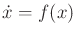is given along with an equilibrium point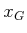. Let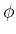denote a candidate Lyapunov function, which will be used as an auxiliary device for establishing the stability of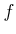. An appropriatemust be determined for the particular vector field. This may be quite challenging in itself, and the details are not covered here. In a sense, the procedure can be characterized as guess and verify,'' which is the way that many solution techniques for differential equations are described. Ifsucceeds in establishing stability, then it is promoted to being called a Lyapunov function for.

It will be important to characterize howvaries in the direction of flow induced by. This is measured by the Lie derivative,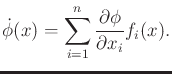(15.3)

This results in a new function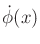, which indicates for eachthe change inalong the direction of.

Several concepts are needed to determine stability. Let a function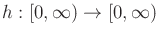be called a hill if it is continuous, strictly increasing, and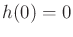. This can be considered as a one-dimensional navigation function, which has a single local minimum at the goal, 0. A function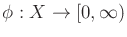is called locally positive definite if there exists some open set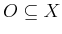and a hill function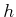such that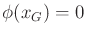and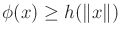for all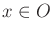. If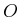can be chosen as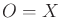, and ifis bounded, thenis called globally positive definite or just positive definite. In some spaces this may not be possible due to the topology of; such issues arose when constructing navigation functions in Section 8.4.4. Ifis unbounded, thenmust additionally approach infinity as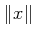approaches infinity to yield a positive definite. For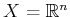, a quadratic form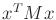, for whichis a positive definite matrix, is a globally positive definite function. This motivates the use of quadratic forms in Lyapunov stability analysis.

The Lyapunov theorems can now be stated [156,846]. Suppose thatis locally positive definite at. If there exists an open setfor which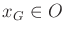, and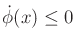on all, thenis Lyapunov stable. If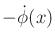is also locally positive definite on, thenis asymptotically stable. Ifand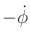are both globally positive definite, thenis globally asymptotically stable.

Example 15..1 (Establishing Stability via Lyapunov Functions)   Let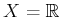. Let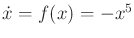, and we will attempt to show that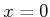is stable. Let the candidate Lyapunov function be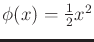. The Lie derivative (15.3) produces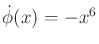. It is clear thatandare both globally positive definite; hence, 0 is a global, asymptotically stable equilibrium point of.Steven M LaValle 2020-08-14